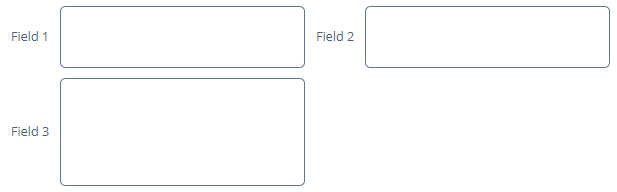# GridLayout

`GridLayout` is a container that allows you to place components in a grid.Component’s XML-name: `gridLayout`.

## Basics

Usage example:

``````<gridLayout spacing="true">
<columns count="4"/>
<rows>
<row>
<label value="Field 1"
align="MIDDLE_LEFT"/>
<textField/>
<label value="Field 2"
align="MIDDLE_LEFT"/>
<textField/>
</row>
<row>
<label value="Field 3"
align="MIDDLE_LEFT"/>
<textField/>
</row>
</rows>
</gridLayout>``````

## Required Elements

### Columns

`columns` – describes grid columns. It should have either a `count` attribute or a nested `column` element for each `column`. It is enough to set the number of columns in the `count` attribute in the simplest case.

If you define the container width in pixels or percents, free space will be divided between the columns equally.

If you define the `flex` attribute for each `column` element, free space will be divided between the columns non-equally.

An example of a `GridLayout` where the second and the fourth columns take all extra horizontal space, and the fourth column takes three times more space:

``````<gridLayout spacing="true"
width="100%">
<columns>
<column/>
<column flex="1"/>
<column/>
<column flex="3"/>
</columns>
<rows>
<row>
<label value="Field 1"
align="MIDDLE_LEFT"/>
<textField width="100%"/>
<label value="Field 2"
align="MIDDLE_LEFT"/>
<textField width="100%"/>
</row>
<row>
<label value="Field 3"
align="MIDDLE_LEFT"/>
<textField width="100%"/>
</row>
</rows>
</gridLayout>``````If the `flex` attribute is not defined or equals `0`, the width of the column will be set according to its contents, given that at least one other column has a non-zero `flex` attribute. In the example above, the first and the third columns will get the width according to the maximum text length.

 For the free space to appear, you should set the entire container width in either pixels or percents. Otherwise, column width will be calculated according to content length, and the `flex` attribute will not affect it.

Attributes of `column`:

### Rows

`rows` − contains a set of rows. Each line is defined in its own `row` element. `row` element can have a `flex` attribute similar to the one defined for the `column` element but affects free vertical space distribution with a given total grid height.

`row` element should contain elements of the components displayed in the grid’s current row cells. The number of components in a row should not exceed the defined number of columns, but it can be less.

For example:

``````<gridLayout spacing="true"
height="200">
<columns count="4"/>
<rows>
<row flex="1">
<label value="Field 1"
align="MIDDLE_LEFT"/>
<textField height="100%"/>
<label value="Field 2"
align="MIDDLE_LEFT"/>
<textField height="100%"/>
</row>
<row flex="2">
<label value="Field 3"
align="MIDDLE_LEFT"/>
<textField height="100%"/>
</row>
</rows>
</gridLayout>``````Any component located in a `GridLayout` can have `colspan` and `rowspan` attributes. These attributes set the number of columns and rows occupied by the corresponding component. For example, this is how the `Field3` `textField` can be extended to cover three columns:

``````<gridLayout spacing="true">
<columns count="4"/>
<rows>
<row>
<label value="Field 1"
align="MIDDLE_LEFT"/>
<textField/>
<label value="Field 2"
align="MIDDLE_LEFT"/>
<textField/>
</row>
<row>
<label value="Field 3"
align="MIDDLE_LEFT"/>
<textField width="100%"
colspan="3"/>
</row>
</rows>
</gridLayout>``````Attributes of `row`: